###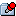Author Topic: [Map Editor] Gamesparks' Map Editor  (Read 20112 times)

#### Knucis

• Jr. Member
•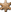• Posts: 50
• Karma: +2/-2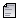##### [Map Editor] Gamesparks' Map Editor
« on: June 26, 2014, 09:19:33 pm »
What is this?
Well, since there is not a map editor for LU (that I know of), I've decided to work on one.
It's extremely simple, nothing too fancy, but does the job.
I didn't test it that much, only with myself (and rarely Thijn) so expect it not being perfect.
So, in simple terms: it's an object placement editor for LU that includes a map loader/saver so you can easily share maps with the community!

Can I have some pictures?What did I just see?
What you saw was the in-game editor. It's quite easy to understand, and you'll be making maps in no time.
The commands are:

Code: [Select]
`/addob <model id> - Creates an object./removeob <object id> - Removes an object./moveob <object id/exit> - Enters the editor./nearob <range> - Messages you all the objects within the range given./savemap <mapname> - Saves all the objects in the world into an XML file (so you can load later)`The editor has 5 control modes:
Code: [Select]
`1 - X/Y object movement.2 - Z object movement.3 - X/Y object rotation.4 - Z object rotation.5 - Camera movement.`It also includes a "sensitivity" features that enables you to control the precision of the movement.

How do I load a custom map?
Alright, for this you need to understand how the XML files work with this script.

Code: [Select]
`<!-- This is the loader file. You can manually load your maps here./--><!-- Just add a new line inside the <loader></loader> with the following code: /--><!--Map name="file name here" /--><loader>    <Map name="example" /></loader>`All it's doing is loading the "example" map. Which in return looks like this:
Code: [Select]
`<example>    <Object model="1356" x="79.34" y="-960.147" z="25.66" rx="0" ry="0" rz="0" world="0" /></example>`So all you have to do to install another map is to edit the LOADER.xml file by adding a new line with the map's name:
Code: [Select]
`<!-- This is the loader file. You can manually load your maps here./--><!-- Just add a new line inside the <loader></loader> with the following code: /--><!--Map name="file name here" /--><loader>    <Map name="example" />    <Map name="mynewmap" /></loader>`You'll need to have the mynewmap.xml file inside the "Maps/" directory and you're good to goMap names must be one word ONLY.

Where do I get it?

How do I install it?
Simply drop everything into your server folder! And then edit your content.xml inside the LU folder.

• If you want to be able to edit maps, load the MapEditor script.
(I highly recommend you not to load the MapEditor on public servers. I didn't add any restrictions. You have been warned.)

Thanks, and please let me know if it has some bugs, I will try to fix themHappy mapping!
« Last Edit: June 27, 2014, 01:01:39 pm by Knucis »

#### Merkel##### Re: [Map Editor] Gamesparks' Map Editor
« Reply #1 on: June 26, 2014, 11:19:38 pm »
Wow! LOL!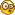Edit: Why not add a message with the object ID when you creates the object?
« Last Edit: June 27, 2014, 01:15:37 am by Merkel »
Westwood Studios

The best studios company in strategy videogames.

#### Knucis

• Jr. Member
•• Posts: 50
• Karma: +2/-2##### Re: [Map Editor] Gamesparks' Map Editor
« Reply #2 on: June 27, 2014, 01:04:05 am »
Edit: Why not add a message with the object ID when is you creates the object?
Will update it tomorrow#### Merkel##### Re: [Map Editor] Gamesparks' Map Editor
« Reply #3 on: June 27, 2014, 01:15:11 am »
Hmm, again problem.

2) When is called 'onPlayerSpawn' by any player gives an error. (The index Pos doesn't exist)
Westwood Studios

The best studios company in strategy videogames.

#### Knucis

• Jr. Member
•• Posts: 50
• Karma: +2/-2##### Re: [Map Editor] Gamesparks' Map Editor
« Reply #4 on: June 27, 2014, 01:37:20 am »
Hmm, again problem.

2) When is called 'onPlayerSpawn' by any player gives an error. (The index Pos doesn't exist)

1) How does it fail? Be more specific. Works fine here...
2) What the hell? I don't even call onPlayerSpawn on my script!

#### Merkel##### Re: [Map Editor] Gamesparks' Map Editor
« Reply #5 on: June 27, 2014, 02:12:53 am »
Hmm, again problem.

2) When is called 'onPlayerSpawn' by any player gives an error. (The index Pos doesn't exist)

1) How does it fail? Be more specific. Works fine here...
2) What the hell? I don't even call onPlayerSpawn on my script!

2) Yeah, i know, but when I don't load the scripts works... in my PC and the VPS same.
Westwood Studios

The best studios company in strategy videogames.

#### Knucis

• Jr. Member
•• Posts: 50
• Karma: +2/-2##### Re: [Map Editor] Gamesparks' Map Editor
« Reply #6 on: June 27, 2014, 02:18:26 am »
Hmm, again problem.

2) When is called 'onPlayerSpawn' by any player gives an error. (The index Pos doesn't exist)

1) How does it fail? Be more specific. Works fine here...
2) What the hell? I don't even call onPlayerSpawn on my script!

2) Yeah, i know, but when I don't load the scripts works... in my PC and the VPS same.
1) How does your Maps/ directory looks like (and what's inside the LOADER.xml file)

#### Merkel##### Re: [Map Editor] Gamesparks' Map Editor
« Reply #7 on: June 27, 2014, 03:31:09 am »
Hmm, again problem.

2) When is called 'onPlayerSpawn' by any player gives an error. (The index Pos doesn't exist)

1) How does it fail? Be more specific. Works fine here...
2) What the hell? I don't even call onPlayerSpawn on my script!

2) Yeah, i know, but when I don't load the scripts works... in my PC and the VPS same.
1) How does your Maps/ directory looks like (and what's inside the LOADER.xml file)

1) XML with the objects. In LOADER.xml the map (the name of the xml)
Westwood Studios

The best studios company in strategy videogames.

#### Knucis

• Jr. Member
•• Posts: 50
• Karma: +2/-2##### Re: [Map Editor] Gamesparks' Map Editor
« Reply #8 on: June 27, 2014, 03:35:57 am »
1) XML with the objects. In LOADER.xml the map (the name of the xml)
Mind posting the contents of both please (map.xml and LOADER.xml)? So I can test it on my end...

#### Merkel##### Re: [Map Editor] Gamesparks' Map Editor
« Reply #9 on: June 27, 2014, 03:44:28 am »
1) XML with the objects. In LOADER.xml the map (the name of the xml)
Mind posting the contents of both please (map.xml and LOADER.xml)? So I can test it on my end...

Code: [Select]
`<Crime City>    <Object model="1696" x="-1622.62" y="-785.8" z="2.98" rx="3" ry="1" rz="32" world="0" />    <Object model="1696" x="-1626.64" y="-733.075" z="4.775" rx="0.75" ry="358.5" rz="34" world="0" />    <Object model="3146" x="-1634.86" y="-652.25" z="10.975" rx="1" ry="359.5" rz="302.5" world="0" />    <Object model="724" x="-1556.45" y="-1009.55" z="10.965" rx="0" ry="0" rz="0" world="0" />    <Object model="725" x="-1281.63" y="-976.95" z="8.59" rx="0" ry="0" rz="0" world="0" />    <Object model="822" x="-1359.8" y="-906.5" z="12.495" rx="359" ry="359.5" rz="196" world="0" />    <Object model="952" x="-1626.52" y="-1087.6" z="16.84" rx="0" ry="0" rz="356" world="0" />    <Object model="1033" x="-1575.38" y="-1038.1" z="10.84" rx="359.75" ry="0.25" rz="0" world="0" />    <Object model="1393" x="-1584.8" y="-957.2" z="10.84" rx="357.5" ry="0" rz="172.5" world="0" />    <Object model="1393" x="-1584.8" y="-988.55" z="10.84" rx="0" ry="0" rz="178.625" world="0" />    <Object model="1347" x="-1548.8" y="-948.8" z="10.53" rx="0" ry="0" rz="0" world="0" />    <Object model="1347" x="-1548.8" y="-944.8" z="10.61" rx="0" ry="0" rz="0" world="0" />    <Object model="1318" x="-1570.88" y="-930.663" z="10.11" rx="0" ry="0" rz="0" world="0" /></Crime City>`
Westwood Studios

The best studios company in strategy videogames.

#### Knucis

• Jr. Member
•• Posts: 50
• Karma: +2/-2##### Re: [Map Editor] Gamesparks' Map Editor
« Reply #10 on: June 27, 2014, 12:53:42 pm »
1) XML with the objects. In LOADER.xml the map (the name of the xml)
Mind posting the contents of both please (map.xml and LOADER.xml)? So I can test it on my end...
It's not working because the map name is "Crime City", aka, has two words. Rename it into one word only please.

EDIT: Rename the Crime City.xml to crimecity.xml, then:
Code: [Select]
`<crimecity>    <Object model="1696" x="-1622.62" y="-785.8" z="2.98" rx="3" ry="1" rz="32" world="0" />    <Object model="1696" x="-1626.64" y="-733.075" z="4.775" rx="0.75" ry="358.5" rz="34" world="0" />    <Object model="3146" x="-1634.86" y="-652.25" z="10.975" rx="1" ry="359.5" rz="302.5" world="0" />    <Object model="724" x="-1556.45" y="-1009.55" z="10.965" rx="0" ry="0" rz="0" world="0" />    <Object model="725" x="-1281.63" y="-976.95" z="8.59" rx="0" ry="0" rz="0" world="0" />    <Object model="822" x="-1359.8" y="-906.5" z="12.495" rx="359" ry="359.5" rz="196" world="0" />    <Object model="952" x="-1626.52" y="-1087.6" z="16.84" rx="0" ry="0" rz="356" world="0" />    <Object model="1033" x="-1575.38" y="-1038.1" z="10.84" rx="359.75" ry="0.25" rz="0" world="0" />    <Object model="1393" x="-1584.8" y="-957.2" z="10.84" rx="357.5" ry="0" rz="172.5" world="0" />    <Object model="1393" x="-1584.8" y="-988.55" z="10.84" rx="0" ry="0" rz="178.625" world="0" />    <Object model="1347" x="-1548.8" y="-948.8" z="10.53" rx="0" ry="0" rz="0" world="0" />    <Object model="1347" x="-1548.8" y="-944.8" z="10.61" rx="0" ry="0" rz="0" world="0" />    <Object model="1318" x="-1570.88" y="-930.663" z="10.11" rx="0" ry="0" rz="0" world="0" /></crimecity>`
Quote
<Map name="crimecity" />
« Last Edit: June 27, 2014, 01:06:18 pm by Knucis »

#### Thijn

• Tester
• Sr. Member
•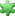• Posts: 526
• Karma: +27/-16##### Re: [Map Editor] Gamesparks' Map Editor
« Reply #11 on: June 27, 2014, 01:02:33 pm »
If you want you can use an underscore (_) to simulate some kind of space.
Something like Crime_City should in theory work.

#### Knucis

• Jr. Member
•• Posts: 50
• Karma: +2/-2##### Re: [Map Editor] Gamesparks' Map Editor
« Reply #12 on: June 27, 2014, 01:04:20 pm »
If you want you can use an underscore (_) to simulate some kind of space.
Something like Crime_City should in theory work.
Yes, underscores should be fine.

#### Merkel##### Re: [Map Editor] Gamesparks' Map Editor
« Reply #13 on: June 27, 2014, 01:11:19 pm »
1) XML with the objects. In LOADER.xml the map (the name of the xml)
Mind posting the contents of both please (map.xml and LOADER.xml)? So I can test it on my end...
It's not working because the map name is "Crime City", aka, has two words. Rename it into one word only please.

EDIT: Rename the Crime City.xml to crimecity.xml, then:
Code: [Select]
`<crimecity>    <Object model="1696" x="-1622.62" y="-785.8" z="2.98" rx="3" ry="1" rz="32" world="0" />    <Object model="1696" x="-1626.64" y="-733.075" z="4.775" rx="0.75" ry="358.5" rz="34" world="0" />    <Object model="3146" x="-1634.86" y="-652.25" z="10.975" rx="1" ry="359.5" rz="302.5" world="0" />    <Object model="724" x="-1556.45" y="-1009.55" z="10.965" rx="0" ry="0" rz="0" world="0" />    <Object model="725" x="-1281.63" y="-976.95" z="8.59" rx="0" ry="0" rz="0" world="0" />    <Object model="822" x="-1359.8" y="-906.5" z="12.495" rx="359" ry="359.5" rz="196" world="0" />    <Object model="952" x="-1626.52" y="-1087.6" z="16.84" rx="0" ry="0" rz="356" world="0" />    <Object model="1033" x="-1575.38" y="-1038.1" z="10.84" rx="359.75" ry="0.25" rz="0" world="0" />    <Object model="1393" x="-1584.8" y="-957.2" z="10.84" rx="357.5" ry="0" rz="172.5" world="0" />    <Object model="1393" x="-1584.8" y="-988.55" z="10.84" rx="0" ry="0" rz="178.625" world="0" />    <Object model="1347" x="-1548.8" y="-948.8" z="10.53" rx="0" ry="0" rz="0" world="0" />    <Object model="1347" x="-1548.8" y="-944.8" z="10.61" rx="0" ry="0" rz="0" world="0" />    <Object model="1318" x="-1570.88" y="-930.663" z="10.11" rx="0" ry="0" rz="0" world="0" /></crimecity>`
Quote
<Map name="crimecity" />

Now works, thx.

And what about the event 'onPlayerSpawn' ?
Westwood Studios

The best studios company in strategy videogames.

#### Knucis

• Jr. Member
•• Posts: 50
• Karma: +2/-2##### Re: [Map Editor] Gamesparks' Map Editor
« Reply #14 on: June 27, 2014, 01:14:01 pm »
And what about the event 'onPlayerSpawn' ?
I have no idea what's causing it, as I said, it might be your script interfering...
MapLoader or MapEditor does not call "onPlayerSpawn" to cause an error.

 © Liberty Unleashed Team. MySQL | PHP | XHTML | CSS